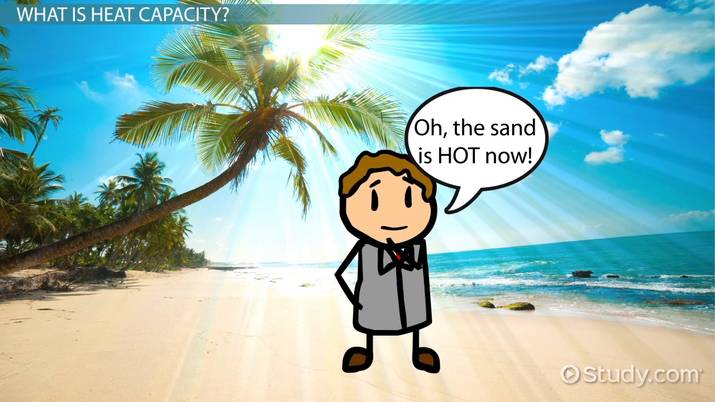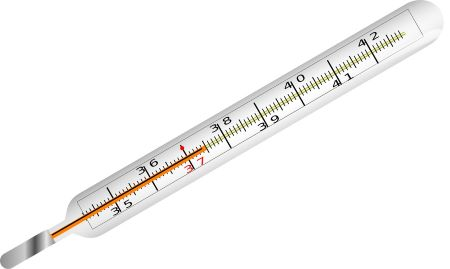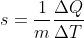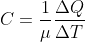Heat Capacity Formula and Examples

Pritha Mandal, Amy Lange, Christianlly Cena
• Author
Pritha Mandal

Pritha is an academic editor and technical writer. She holds a Master's degree in Physics, with specialization in Astrophysics. Pritha has edited hundreds of physical science research papers and has co-authored a paper on radio astronomy.

• Instructor
Amy Lange

Amy has taught university-level earth science courses and has a PhD in Geology.

• Expert Contributor
Christianlly Cena

Christianlly has taught college physics and facilitated laboratory courses. He has a master's degree in Physics and is pursuing his doctorate study.

See the heat capacity definition and symbol. View examples to learn how to use the heat capacity formula to calculate the heat capacity of a substance. Updated: 08/13/2021

Show

What is Heat?

Before investigating the concept of heat capacity, take a quick look at what heat is. Heat is a form of energy that is capable of flowing from one system to another. To understand heat as a transferable energy source, consider the boiling of water. When a vessel filled with water is placed on a burning stove, the bottom of the vessel first heats up, following which the water begins to get heated up until it finally starts boiling. This sequence of heat flow is directly related to the temperature of the vessel and the water; the temperature of a body is a measure of its hotness or coldness and it determines the direction of heat flow.

Thus, for heat energy to flow from one system to another, the two systems must have different temperatures. Heat will then flow from the hotter system to the colder system until the two systems reach the same temperature. Consider a bucket half filled with scorching hot water. To make the water slightly cooler, cold water is added to the bucket. Heat from the hot water makes the cold water warmer, until finally the mixture is at an intermediate temperature. Notably, it is the 'hotness' that flows among systems; 'coldness' is just the absence of heat.

As heat is a form of energy, it is measured in Joules (J) in the SI system and in calories (cal) in the CGS system.

{eq}1\:cal=4.184\:J {/eq}

Internal Energy

In simple words, the internal energy of a system is the sum of the kinetic and potential energies of the molecules constituting the system. The molecules of a system exhibit random, chaotic motion, which results in the internal energy of the system. The internal energy of a system is closely related to its temperature. The more energetic the motion of the molecules, the higher is the internal energy of the system, and consequently, the higher is the corresponding temperature.An error occurred trying to load this video.

Try refreshing the page, or contact customer support.

Coming up next: Heat of Fusion: Definition, Equation & Examples

You're on a roll. Keep up the good work!

Replay
Your next lesson will play in 10 seconds
• 0:00 What Is Heat Capacity?
• 0:35 Understanding Heat
• 1:35 Defining Heat Capacity
• 3:05 Specific Heat
• 3:45 Lesson Summary
Save Save

Want to watch this again later?

Timeline
Autoplay
Autoplay
Speed Speed

Heat Capacity Definition

Now that the concepts of heat and internal energy have been defined, what is heat capacity? The heat capacity of a substance is the amount of heat energy required to raise its temperature by one unit. Heat capacity is thus an inherent property of a substance. For example, water has an extremely high heat capacity of 4184 J per kilogram. This implies that 4184 J of heat energy is needed to raise the temperature of water by 1 Kelvin (or 1 Celsius).Effect of Heat

A very interesting effect that heat has on solid objects is the expansion of the dimensions of the object. This phenomenon is called thermal expansion and it can be of three kinds.

1. Expansion of the length of the object or linear expansion
2. Expansion of the area of the object or area expansion
3. Expansion of the volume of the object or volume expansionDifference Between Heat Capacity, Specific Heat Capacity, and Molar Heat Capacity

The heat capacity of a substance does not consider the amount of the substance. When the amount of the substance is also considered, the concepts of specific heat capacity and molar heat capacity are used.

Specific Heat Capacity

The specific heat capacity of a substance is defined as the heat required to change the temperature of unit mass of the substance by one unit. The following expression is used to mathematically define the specific heat capacity.Here, {eq}\Delta Q {/eq} is the amount of heat supplied, {eq}\Delta T {/eq} is the temperature change, and {eq}m {/eq} is the mass of the substance.

The SI unit of specific heat capacity is Joules per kilogram per Kelvin ({eq}Jkg^{-1}K^{-1} {/eq}.

Molar Heat Capacity

Gaseous substances are usually expressed in terms of moles ({eq}\mu {/eq}) rather than mass. Thus, for gases, the molar heat capacity is generally used. Molar heat capacity is defined as the heat required to change the temperature of unit mole of the gaseous substance by one unit. It is given by:The SI unit of molar heat capacity is Joules per mole per Kelvin ({eq}Jmol^{-1}K^{-1} {/eq}. Molar heat capacity can be defined at constant pressure ({eq}C_{p} {/eq}) or at constant volume of the gas ({eq}C_{v} {/eq}).

Consider the following table to understand the differences between heat capacity, specific heat capacity, and molar heat capacity.

Heat Capacity Specific Heat Capacity Molar Heat Capacity
1. It is the heat required for unit temperature change. 1. It is the heat required per unit mass for unit temperature change. 1. It is the heat required per mole for unit temperature change.
2. It does not depend on the quantity of the substance. 2. It depends on the mass of the substance. 2. It depends on the number of moles of the substance.
3. For example, water has a high heat capacity implies that a high amount of heat energy is required to raise the temperature of water by a certain degree. 3. For example, the specific heat capacity of water is 4184 J/(kg.K) implies that 4184 J of heat energy is required to raise the temperature of 1 kg water by 1 K. 3. For example, the molar heat capacity of water (at constant pressure) is 75 J/(mol.K) implies that 75 J of heat energy is required to raise the temperature of 1 mole of water by 1 K.

In general, the specific heat capacity of substances is used to represent how much heat they require for changing their temperature. The table below provides the specific heat capacity values for some common substances.

To unlock this lesson you must be a Study.com Member.

• Activities
• FAQs

Heat Capacity True or False Activity

Directions

Determine whether the following statements are true or false. To do this, print or copy this page on a blank paper and underline or encircle the answer.

1. Specific heat denotes the amount of heat required to raise the temperature of a substance per unit of mass.

True | False

2. Sand has a much higher capacity for storing heat energy than water.

True | False

3. Internal energy is defined as the energy associated with the random, disordered motion of molecules within an object.

True | False

4. The amount of heat required to change the temperature of water (with a heat capacity of 400 Joules/degree Celsius) by 30 degrees Celsius is 1200 Joules.

True | False

5. Heat energy is transferred from a body of lower temperature to those with a higher temperature

True | False

6. In the same sunlight, the temperature of water increases more slowly than the temperature of the sand.

True | False

7. Different substances have different capacities for storing heat energy.

True | False

8. The amount of heat, Q, is directly proportional to mass and resultant temperature.

True | False

9. Heat capacity is defined as the amount of energy required to increase the temperature of a specific substance by 10 degrees Celsius.

True | False

10. In the International System of Units, Celsius is the derived unit of energy, work, and heat.

True | False

1. True
2. False, because the correct statement is, Water has a much higher capacity for storing heat energy than sand.
3. True
4. False, because the correct statement is, The amount of heat required to change the temperature of water (with a heat capacity of 400 J / degree Celsius) by 30 degrees Celsius is 12000 Joules.
5. False, because the correct statement is, Heat energy is transferred from a body of higher temperature to those with a lower temperature.
6. True
7. True
8. True
9. False, because the correct statement is, Heat capacity is defined as the amount of energy required to increase the temperature of a specific substance by 1 degree Celsius..
10. False, because the correct statement is, In the International System of Units, Joule is the derived unit of energy, work, and heat.

How do you calculate heat capacity in thermodynamics?

In thermodynamics, the heat capacity is a measure of the heat required by a substance to raise its temperature by a specific degree. The heat capacity is defined as the amount of heat that is needed for a unit rise in the temperature of the substance.

What is heat capacity equal to?

The heat capacity of a substance (S) is equal to the ratio of the amount of heat energy supplied to the substance (Delta Q) to the corresponding rise in temperature (Delta T).

S=Delta Q/Delta T

What determines heat capacity?

The heat capacity is determined by the quantity of heat energy supplied to the concerned substance. It is also dependent on the initial and final temperatures of the substance.

What is the SI unit of heat capacity?

The heat capacity of a substance is the ratio between the heat supplied to the substance and the corresponding temperature change. Thus, the SI unit of heat capacity is Joules per Kelvin (J/K).

Register to view this lesson

Are you a student or a teacher?